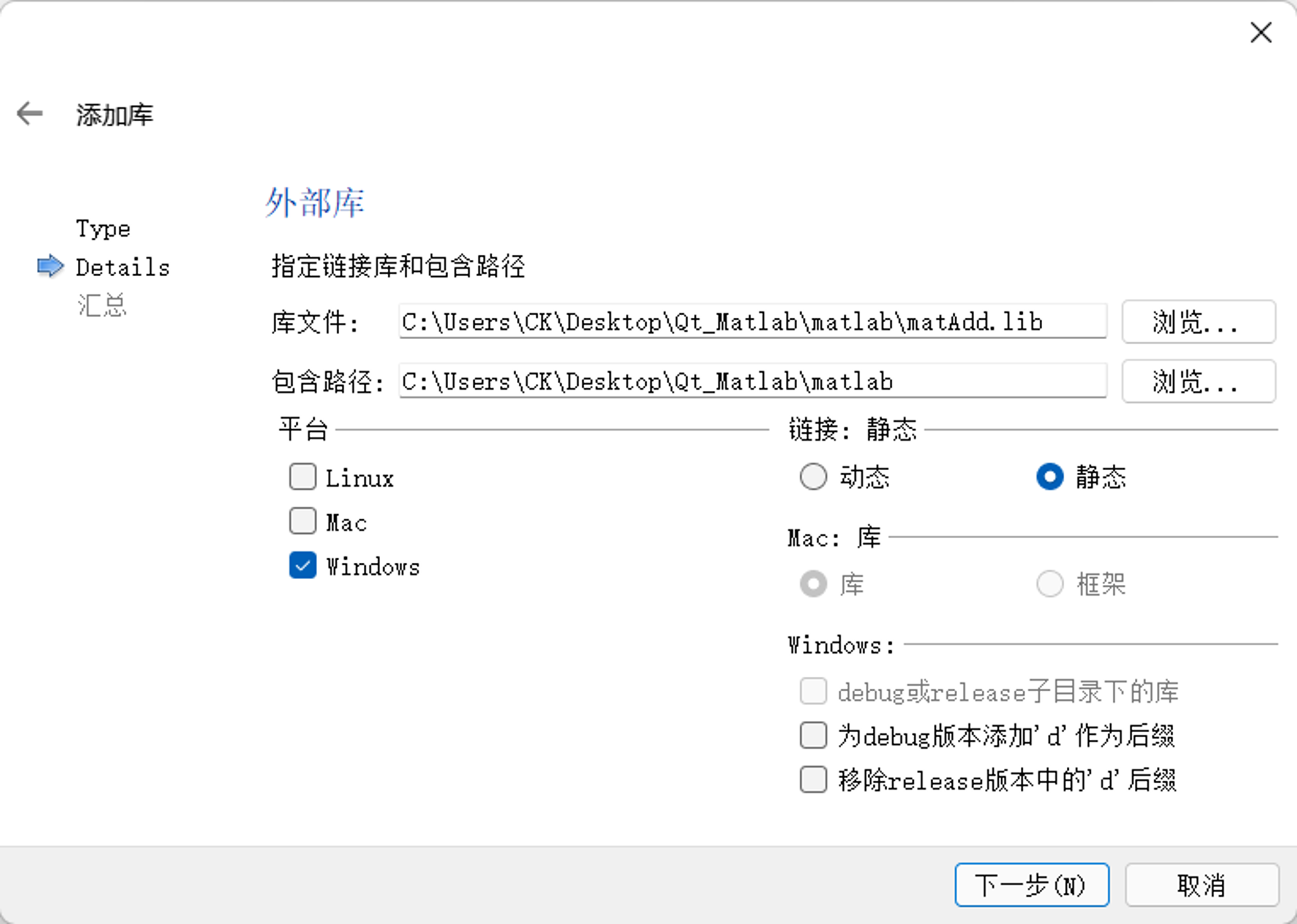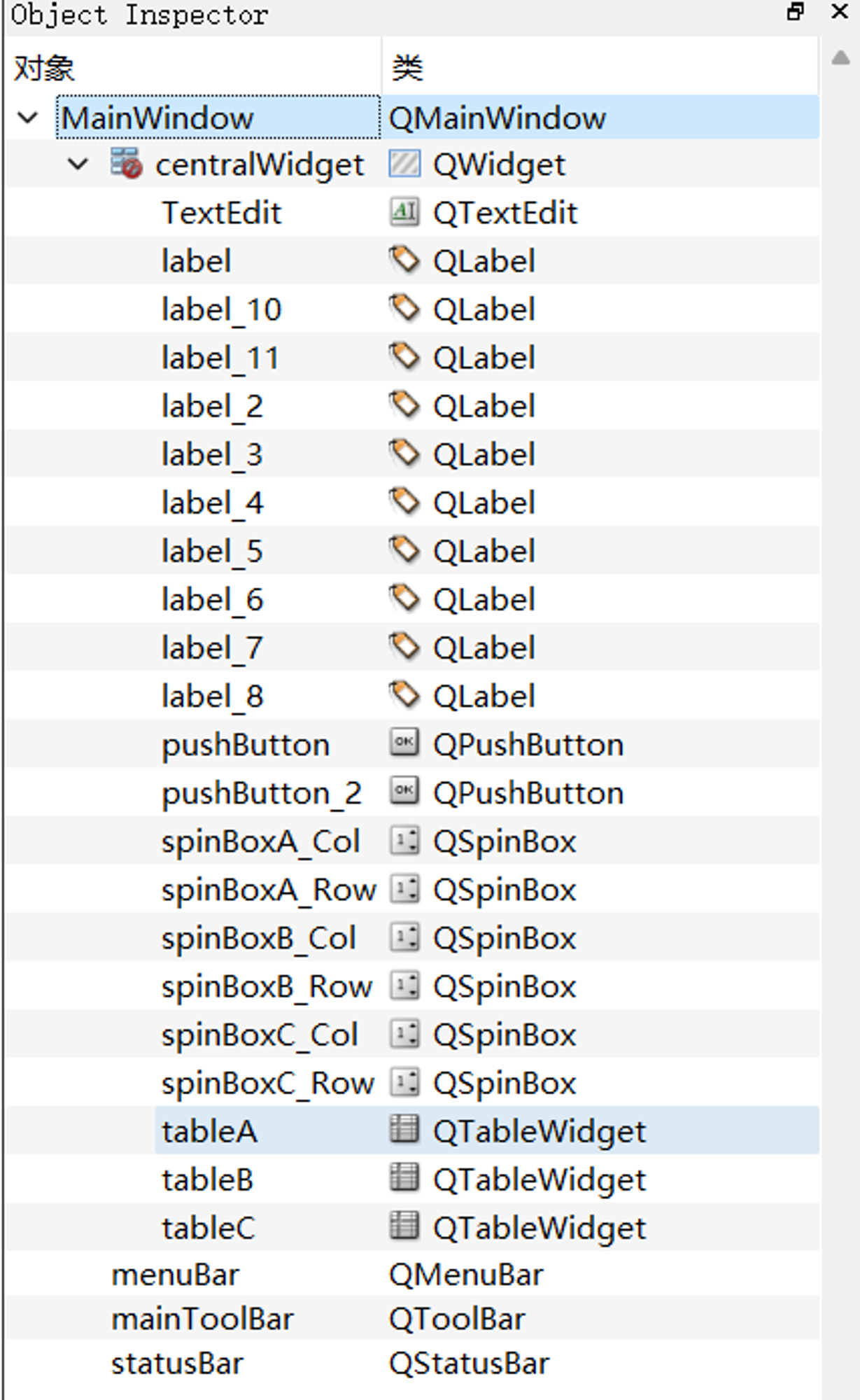K

Kern

V1

2023/03/09阅读：31主题：橙心

# Qt C++ Matlab 混合编程测试使用文档

## 环境版本

• Qt：5.9.0
• MSVC 2017 64bit 编译器
• Matlab：R2019b （64bit）
• Windows 11 64位

## 开发步骤

• 如何在Matlab中将m文件编译为C++语言的DLL文件
• 如何在Qt项目中加入自定义DLL相关的LIB文件，以及MATLAB相关的LIB文件和H文件搜索路径
• 如何在Qt中调用自定义DLL中的函数，如何通过mwArray类传递输入输出参数

### Matlab 动态链接库的生成

#### Matlab 函数的编写

``function  C= matAdd(A,B)% C= matAdd(A,B),  两个矩阵相加C=A+B;end``

#### 设置MATLABCompiler 编译器

``>>  mbuild -setup C++MBUILD 配置为使用 'Microsoft Visual C++ 2017' 以进行 C++ 语言编译。``

#### Library Compiler

``>> deploytool``‘TYPE’ 部分选择 C++ Shared Library ，“EXPORTED FUNCTIONS” 选择待添加的文件（这里可以添加多个函数文件）。右侧是MATLAB运行时库的安装打包方式，在本机上测试可选择“Runtime downloaded from web”。添加完毕后点击‘Package’ 进行编译和打包。

### Qt 调用环境配置``win32: LIBS += -L\$\$PWD/./ -lmatAdd    INCLUDEPATH += \$\$PWD/.DEPENDPATH += \$\$PWD/.win32:!win32-g++: PRE_TARGETDEPS += \$\$PWD/./matAdd.libelse:win32-g++: PRE_TARGETDEPS += \$\$PWD/./libmatAdd.a``

#### Matlab 依赖库和头文件搜索路径的加入。

``INCLUDEPATH += 'D:/Program/matlab2019/matlab/extern/include'INCLUDEPATH += 'D:/Program/matlab2019/matlab/extern/include/win64'win32: LIBS += -L'D:/Program/matlab2019/matlab/extern/lib/win64/microsoft/' -llibmexwin32: LIBS += -L'D:/Program/matlab2019/matlab/extern/lib/win64/microsoft/' -llibmxwin32: LIBS += -L'D:/Program/matlab2019/matlab/extern/lib/win64/microsoft/' -llibmatwin32: LIBS += -L'D:/Program/matlab2019/matlab/extern/lib/win64/microsoft/' -llibengwin32: LIBS += -L'D:/Program/matlab2019/matlab/extern/lib/win64/microsoft/' -lmclmcrwin32: LIBS += -L'D:/Program/matlab2019/matlab/extern/lib/win64/microsoft/' -lmclmcrrtINCLUDEPATH += 'D:/Program/matlab2019/matlab/extern/lib/win64/microsoft'DEPENDPATH += 'D:/Program/matlab2019/matlab/extern/lib/win64/microsoft'INCLUDEPATH += 'D:/Program/matlab2019/matlab/extern/lib/win64'DEPENDPATH += 'D:/Program/matlab2019/matlab/extern/lib/win64'``

#INCLUDEPATH+=\$\$quote(D:/MATLAB2017b/extern/include)

#### 系统环境变量的配置

``>> mcrinstaller``

### C++ 对 Matlab 函数调用``MainWindow::MainWindow(QWidget *parent) :    QMainWindow(parent),    ui(new Ui::MainWindow){    ui->setupUi(this);    // 不执行matAddInitialize()会报错，注意matAdd为文件名    if(matAddInitialize())    {        ui->TextEdit->setText("matlab程序DLL初始化成功.");    }    else    {        ui->TextEdit->setText("*** matlab程序DLL初始化失败");        return;    }}``

#### mwArray 类的使用

（1）构造函数如下所示：

``   mwArray(num_rows,num_cols,mxID,cmplx=mxREAL)``
• num_rows表示行数， mwSize是整数类型

• num_cols表示列数

• mxID是mxClassID类型，表示元素的基本数据类型，常见的有如下的一些取值mxLOGICAL_CLASS

mxCHAR_CLASS

mxDOUBLE_CLASS

mxSINGLE_CLASS

mxINT8_CLASS

mxUINT8_CLASS

mxINT16_CLASS

mxUINT16_CLASS

mxINT32_CLASS

mxUINT32_CLASS

mxINT64_CLASS

mxUINT64_CLASS

• cmplx是mxComplexity类型，有mxREAL和mxCOMPLEX两种取值，标书数组元素是实数或复数，缺省为mxREAL

（2）mwArray数组的赋值

``mwArray:: SetData(mxUint64* buffer, mwSizelen) ``

``void MainWindow::on_pushButton_clicked(){// 两个矩阵相加， C=A+B//读取矩阵A       int   rowCntA=ui->spinBoxA_Row->value();       int   colCntA=ui->spinBoxA_Col->value();       int   elementCntA=rowCntA*colCntA; //元素个数       //一维数组，用于C++向 MATLAB数组传递数据       double   *arrayA=new double[elementCntA];        int N=0; //C++的一维数组的元素索引号          for(int i=0;i<ui->tableA->columnCount();i++) //逐列读取，序列化存储到一维数组             for (int j=0; j<ui->tableA->rowCount();j++)             {                arrayA[N]=ui->tableA->item(j,i)->text().toDouble();                N++;             }         //定义数组，行，列，double类型          mwArray matrixA(rowCntA,colCntA,mxDOUBLE_CLASS, mxREAL);         //将C++ 的一维数组arrayA存储到 MATLAB的二维数组matrixA          matrixA.SetData(arrayA,elementCntA); ``

（3）mwArray数组元素的读取

``int dim=2; // 二维数组double   value=matrixA.Get(dim,j,i); //按照dim维数数组读出，第j行， 第i列``

``int dim=2; // 二维数组double   value=matrixA.Get(dim,N); //按照dim维数数组读出，第N个元素``

``   double   value=matrixC(j,i); //直接用数组下标索引，第j行，第i列   double   value=matrixC(N); //直接按元素序号读取, 第N个元素``

#### Matlab 函数的调用

• nargout：输出变量个数
• matrixC: 保存返回矩阵的 mwArray类，需要指定相关属性。
• matrixA、matrixB：matlab 原函数中的两个参数矩阵。
``          //计算, C=A+B            int   rowCntC=rowCntA;            int   colCntC=colCntA;  //定义数组，行，列，double类型             mwArray matrixC(rowCntC,colCntC,mxDOUBLE_CLASS, mxREAL);             int nargout=1;//输出变量个数  // 调用函数，计算 C = A + B             matAdd(nargout,matrixC,matrixA,matrixB);``

## 其他官方文档及常见问题

### mwArray 类官方文档

Class used to pass input/output arguments to C++ functions generated by MATLAB Compiler SDK

### 常见问题

Qt与Matlab混合编程细节总结

K
V1